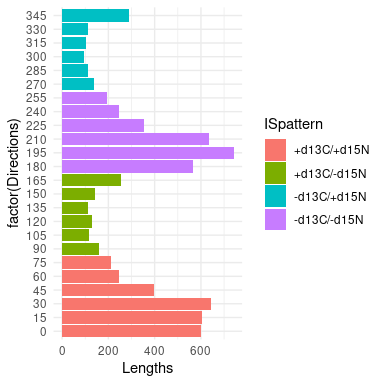# Stable Isotope Trajectory Analysis (SITA)

#### 2022-04-26

This vignette present some ecological applications used by Sturbois et al. (2021) in the new stable isotope trajectory analysis framework:

• Sturbois, et al., Stable Isotope Trajectory Analysis (SITA): A new approach to quantify and visualize dynamics in stable isotope studies. Ecol. Monogr. (2021). https://doi.org/10.1002/ecm.1501

Example datasets have been included in the package for reproducibility. After the calculation of the necessary distance- and direction-based metrics, the vignette focuses, for each ecological applications, on the creation of trajectory charts.

First of all, we load the required libraries, including ecotraj:

## NOTE: Either Arial Narrow or Roboto Condensed fonts are required to use these themes.
##       Please use hrbrthemes::import_roboto_condensed() to install Roboto Condensed and
##       if Arial Narrow is not on your system, please see https://bit.ly/arialnarrow
## Loading required package: viridisLite
##
## Attaching package: 'viridis'
## The following object is masked from 'package:scales':
##
##     viridis_pal

## 3. Spatial and temporal resource partitioning in fur seals

### 3.1 Fur seal stable isotope dataset

We begin by loading the package dataset furseals:

data("furseals")

This is a subset of the dataset provided in:

• Kernaléguen, L., Arnould, J.P.Y., Guinet, C., Cherel, Y., 2015. Determinants of individual foraging specialization in large marine vertebrates, the Antarctic and subantarctic fur seals. Journal of Animal Ecology 1081–1091.

Briefly, fur seals [the Antarctic fur seal Arctocephalus gazella (AFS) and subantarctic fur seal A. tropicalis (SAFS)] whisker SI values yield unique long-term information on individual behaviour which integrates the spatial, trophic and temporal dimensions of the ecological niche. The foraging strategies of this two species of sympatric fur seals were examined in the winter 2001/2002 at Crozet, Amsterdam and Kerguelen Islands (Southern Ocean) using the stable isotope values of serially sampled whiskers. The method consists in the analysis of consecutive whisker sections (3 mm long) starting from the proximal (facial) end, with the most recently synthesized tissue remaining under the skin. Only individuals (n = 47) with whiskers totalizing at least 30 sections were selected in the initial data, and only those 30 sections were considered herein, from t1 (more recent values) to t30 (oldest values).

### 3.2 Trajectory metrics

In this section, we illustrate how to calculate trajectory metrics to characterize the foraging strategy of each fur seal. In the following sections, we show how to use these metrics as data to create plots.

First, we calculate net changes relative to the initial state (i.e. the distance between stable isotope compositions (i.e state) of each whisker section and the initial stable isotope composition):

Net_changes<-trajectoryLengths2D(furseals[,c("d13C","d15N")],
furseals$ID_SITA, furseals$Time, relativeToInitial=TRUE)
head(Net_changes)
##       Lt1_t2    Lt1_t3    Lt1_t4    Lt1_t5     Lt1_t6    Lt1_t7    Lt1_t8
## 1 0.08246211 1.0892314 2.0775851 2.3714985 2.20626585 1.7839633 1.6819920
## 2 0.59304131 0.9583074 0.8218643 0.2677928 0.08105554 0.7460168 0.4970191
## 3 0.23256182 1.4622975 3.0029069 2.8817373 2.55906428 1.6445066 1.6734124
## 4 1.24068086 2.1744498 2.3520872 2.4028260 2.28455422 2.2679323 0.9493714
## 5 1.80624915 3.0818595 3.8011940 4.4793800 4.50949775 4.3885128 2.9507619
## 6 1.13903687 4.3444610 4.0299700 6.4151503 5.96779901 6.0314152 7.2216151
##     Lt1_t9   Lt1_t10   Lt1_t11   Lt1_t12   Lt1_t13   Lt1_t14   Lt1_t15
## 1 1.344206 1.2735702 1.0631895 0.7915611 0.8486489 1.0500638 1.1961910
## 2 1.092157 0.6772156 0.7744366 0.2702240 0.6136685 1.0261861 0.7000864
## 3 2.518128 2.0782324 1.7294097 1.1594387 1.7649975 2.7768920 2.4641059
## 4 0.577084 0.6049504 0.5920490 1.5973206 1.1985112 0.8119858 0.6936173
## 5 2.187465 0.9662236 2.6143697 2.7163971 2.7958673 2.6837384 2.2504011
## 6 7.173525 6.2867554 0.2478810 0.9972668 0.9076795 0.5088025 0.6640821
##     Lt1_t16   Lt1_t17   Lt1_t18   Lt1_t19  Lt1_t20  Lt1_t21   Lt1_t22   Lt1_t23
## 1 1.5432880 1.6484832 1.6042908 1.6566572 1.108358 0.381577 0.3006742 1.4772136
## 2 0.5592397 0.4460235 0.6495922 0.7966461 1.787011 1.591433 1.5776276 1.6305889
## 3 0.2755014 0.4497755 0.6170292 0.2879392 1.103359 2.145421 2.1758332 2.1139858
## 4 0.3626927 0.7276160 0.9288983 0.7955941 2.352899 1.851222 2.2158782 2.3341939
## 5 1.8418754 1.8304906 1.8207087 1.5938494 1.553721 1.583789 1.5700115 0.6566011
## 6 3.6309179 4.0313501 6.0026223 6.9996921 6.341455 7.297884 7.5324814 6.1068566
##     Lt1_t24   Lt1_t25   Lt1_t26   Lt1_t27    Lt1_t28   Lt1_t29   Lt1_t30
## 1 1.5890755 1.0783103 1.1172381 2.0638181 2.52046504 2.2934779 1.6320787
## 2 0.2046069 0.3937309 0.3849480 0.7032112 0.89491061 0.3901513 0.7480241
## 3 2.2036883 1.8413856 1.6354464 1.9473328 1.59052601 1.3147125 1.6315076
## 4 2.1019596 0.2814321 0.4237924 0.7473988 0.05360037 0.3889730 0.7375269
## 5 4.0823528 4.4187248 4.3025323 4.3520214 3.43035873 0.7908375 1.2435373
## 6 1.7282780 2.1873694 1.2698965 0.5391030 0.40993902 0.4085156 0.7709643
##   Trajectory
## 1   40.87543
## 2   21.87682
## 3   49.28114
## 4   36.05110
## 5   76.30333
## 6  107.19276

We then calculate trajectory segment lengths, i.e. the distance between the stable isotope composition of consecutive whisker sections in the stable isotope space:

Segment_lengths<-trajectoryLengths2D(furseals[,c("d13C","d15N")],
furseals$ID_SITA, furseals$Time, relativeToInitial=FALSE)
head(Segment_lengths)
##           S1        S2       S3         S4         S5         S6        S7
## 1 0.08246211 1.0799190 1.109759 0.39708060 0.16604819 0.69542793 0.1332817
## 2 0.59304131 0.4019664 0.165463 0.62878295 0.24977790 0.81093588 0.4377728
## 3 0.23256182 1.2719328 1.561554 0.13206059 0.81044432 1.21893232 0.3748826
## 4 1.24068086 0.9337907 0.177792 0.08732125 0.11957006 0.06462971 1.3506817
## 5 1.80624915 1.2835334 1.452408 1.22947021 0.05423099 0.33640006 1.4518034
## 6 1.13903687 3.2697465 1.007842 2.40400083 1.02618176 0.80004000 1.4924584
##           S8         S9       S10       S11        S12       S13       S14
## 1 0.35206960 0.13800362 0.2119929 0.3940774 0.08004998 0.2026154 0.2040221
## 2 0.94680304 0.44818858 0.6290191 0.5362695 0.52697249 0.4552637 0.3411231
## 3 1.04072379 0.44107256 0.3592548 0.7240691 0.66598649 1.0131856 0.4798427
## 4 1.44655867 0.09009439 1.1117198 2.1338287 0.39901128 1.4341130 0.3064376
## 5 0.78147681 1.29231962 3.5747035 0.6261150 0.09265528 0.2502838 0.6440194
## 6 0.05115662 0.88680381 6.3555849 0.9913889 0.09047099 0.4029814 1.1639300
##         S15       S16       S17       S18       S19       S20        S21
## 1 0.3703039 0.1632330 1.7046178 1.0355916 1.0132186 0.7423544 0.11970798
## 2 0.1554670 0.2837781 0.2257632 0.6603492 0.9904383 0.5893293 0.03956008
## 3 2.3198028 0.5979908 0.3078262 0.3993219 1.3487198 1.1301832 0.04002499
## 4 0.8420291 0.4612776 1.5254799 0.1874727 1.5712829 0.5036268 0.41386471
## 5 0.4404407 0.5163768 0.5204652 0.2380084 0.1802276 0.2205108 0.22402009
## 6 2.9863858 0.7196339 1.9833855 1.0789699 0.7247068 0.9616637 0.24162988
##          S22       S23       S24       S25       S26       S27       S28
## 1 1.76281735 0.1147911 0.6148642 0.3162910 0.9585927 0.5338661 0.2521686
## 2 0.27828942 1.4322639 0.5912495 0.7604426 0.3286716 0.2085977 0.5259743
## 3 0.09244458 0.1509503 0.6998436 0.3191520 0.6441894 0.5047336 0.3805181
## 4 0.25223997 0.2500180 1.8461901 0.6878982 0.3330540 0.7518151 0.3540353
## 5 2.03381243 3.4923735 0.4153228 0.1254313 0.1696408 0.9698892 2.7246227
## 6 1.48631692 7.3792361 0.6324634 0.9520021 0.9154939 0.1794937 0.3834071
##         S29 Trajectory
## 1 0.6836988   15.63293
## 2 0.3593800   14.60093
## 3 0.3359345   19.59814
## 4 0.5050881   21.38160
## 5 1.8207584   28.96757
## 6 0.9149454   42.62136

Finally, we determine the angle ($$\alpha$$) of consecutive trajectory segments with respect to the second axis of the 2D stable isotope space:

Angles<-trajectoryAngles2D(furseals[,c("d13C","d15N")],
furseals$ID_SITA, furseals$Time, betweenSegments=FALSE)
head(Angles)
##    Axis2-t1  Axis2-t2  Axis2-t3   Axis2-t4   Axis2-t5  Axis2-t6  Axis2-t7
## 1  75.96376 161.64894 195.57662 222.448400   9.008504  48.14751  25.79603
## 2 179.32369 147.49601 315.00000 337.758643  47.109090 356.04023 139.72521
## 3 243.98585 206.02112 198.13327   1.735705 326.780524  10.11145 151.99944
## 4  64.19912  63.68191  66.46289 119.500725 237.639062 171.99527 255.90203
## 5 263.32352 271.65188 195.28972 181.584670 174.709919 343.77386  61.26355
## 6  65.68510  43.26505 163.63292  53.082435 298.712949 139.46085  99.02053
##    Axis2-t8  Axis2-t9 Axis2-t10   Axis2-t11 Axis2-t12 Axis2-t13 Axis2-t14
## 1 358.86075 283.83485 352.13745  22.5303928 192.99462 157.35838 199.76717
## 2 307.01513 171.27328 215.35767  80.5552424  19.74159  26.62133 201.67665
## 3 186.01189  37.90916  45.90222   0.7913279 198.10848 214.09786  81.85301
## 4 250.78846 351.06043  81.67311 261.0762308  90.43079  53.84926 234.02761
## 5  62.15609  60.16163  36.43393 125.7717105  83.18179 293.79978 270.44483
## 6 265.51539 245.97847 247.59927 224.5913352  66.55194  64.26158  61.40918
##   Axis2-t15 Axis2-t16 Axis2-t17 Axis2-t18 Axis2-t19 Axis2-t20 Axis2-t21
## 1 182.32153 112.32286  37.13207  351.0561 280.57797 209.98421 149.36458
## 2 203.10181 122.62605  56.38032  290.3834 341.27235 223.14352  73.85566
## 3  34.05313  18.22286 331.68664  173.8183 198.63646 223.85280 167.00538
## 4 180.47632 178.01222  48.64094  208.3422  64.39713 244.35079  38.91926
## 5 236.67073 131.62432 147.71456  228.4064 173.30874 159.83930 179.23269
## 6  52.20915 115.78169  71.69294   42.1451 219.40066  71.88535  52.22900
##   Axis2-t22 Axis2-t23 Axis2-t24 Axis2-t25 Axis2-t26 Axis2-t27 Axis2-t28
## 1 188.15309  198.2771  338.7350 109.58071 196.73359 224.92411 352.48043
## 2  48.05870  155.7613  152.8282 351.45433  23.67929 346.69657 176.51200
## 3  74.31001  150.2080  331.4941 353.34260 156.77805  68.62019 338.89763
## 4 129.36932  270.6875  251.1332 240.37909 268.96776  74.65130 298.88170
## 5 254.83630  245.0713  203.1080  35.59078 163.91542  71.91999  58.09492
## 6 231.11744  239.4211  251.8515  22.74023  21.46438 118.99260 128.75316
##   Axis2-t29
## 1  31.96985
## 2  28.77587
## 3 186.15184
## 4 235.64922
## 5  61.49251
## 6  67.77996

### 3.3 Identification and characterization of trajectory clusters

Here we aim to define groups of fur seals depending on the similarity of their foraging strategy. We need first to calculate distances between pairs of complete trajectories in the stable isotope space:

D <- dist(furseals[,c("d13C","d15N")])
Ds<-trajectoryDistances(D, furseals$ID_SITA, surveys = NULL, distance.type = "DSPD", symmetrization = "mean", add = TRUE, verbose = FALSE) Then, we can use function hclust() to conduct a hierarchiacl clustering on the symmetric matrix D: colstd<-c("black","yellow","green","blue","grey","red") pt<-c(16,16,16,16) hsxy <- hclust(Ds, "ward.D2") plot(hsxy,hang = -1, main="distance Fur Seals", cex=.6) Hst=2 # Cutting height x<-rect.hclust(hsxy, h=Hst, border = colstd)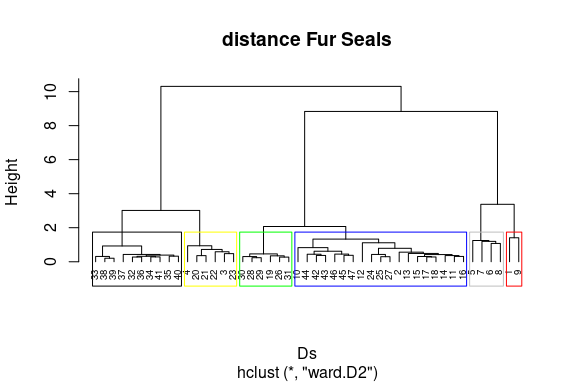We cut the dendrogram at height Hst to obtain a vector of cluster membership and copy it in furseals as a factor: groups <- cutree(hsxy, h=Hst) furseals$cluster <- as.factor(groups)

#### 3.3.1 Individual trophic trajectories for males and females of A. gazella and A. tropicalis

Here we display trophic trajectories of all individuals, in plots corresponding to combinations of species and gender. To facilitate such plots, we create of a vector with the combination of species and gender:

furseals$sp_gender<-paste(furseals$Sexe, furseals$Species, sep=" ") We now create a diagram to display fur seal trophic trajectories in the stable isotope space. Panels correspond to the combination of species and gender. In each panel, X-Y axes are defined by d13C and d15N stable isotope values. Arrows connects all whiskers section SI states from t1 to t30 (i.e. most recent to oldest SI state). Colors corresponds to trajectory clusters and shape to breeding sites: ggplot(data=furseals,aes(x=d13C,y=d15N,color=cluster,shape=Place))+ geom_point()+ geom_path(aes(x=d13C,y=d15N,group=ID_SITA,color=cluster), arrow = arrow(length = unit(0.10, "cm")))+ xlab(expression(delta^13*"C"))+ ylab(expression(delta^15*"N"))+ facet_wrap(~sp_gender) + theme_classic()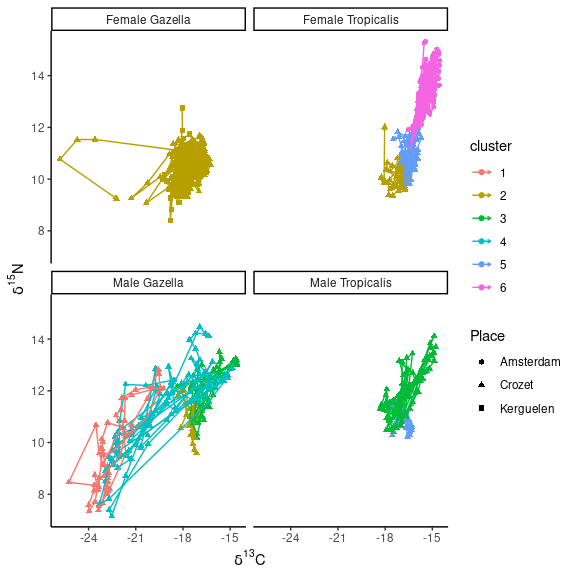#### 3.3.2 Net changes time series for males and females of both fur seal species In this sub-section we display net changes time series for all individuals, in plots corresponding to combinations of species and gender We prepare a subset of the data called NC: NC<-Net_changes[,-30] NC$cluster<-furseals$cluster[1:47] NC$ID<-as.numeric(rownames(NC))
colnames(NC)<-c(2:30,"cluster","ID")

We then prepare the subset. We notably transform NC to a longer format, order the data set and add the vector sp_gender:

NCline <- tidyr::pivot_longer(NC, 1:29,
names_to ="Time_from_present",
values_to="Net_changes",
names_transform = function(x) {as.numeric(x)-1})
colnames(NCline)[1:2]<-c("Clusters", "ID")
NCline <- NCline[order(NCline$Time_from_present, decreasing=FALSE),] NCline <- as.data.frame(NCline) NCline$sp_gender<-c(furseals$sp_gender[1:47]) We now create the plot to display net changes time series for all individuals in panel corresponding Arrows connects all whiskers section stable isotope values from t1 to t30 (i.e. most recent to oldest stable isotope values). Colours corresponds to trajectory clusters: ggplot(data=NCline,aes(x=Time_from_present,y=Net_changes,color=Clusters))+ geom_path(aes(x=Time_from_present,y=Net_changes,group=ID,color=Clusters), arrow = arrow(length = unit(0.10, "cm")))+ facet_wrap(~sp_gender)+ theme_classic()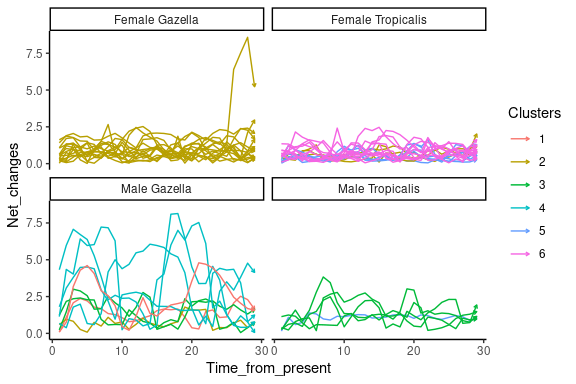#### 3.3.3 Angle $$\alpha$$ trajectory roses of Fur seals trajectory cluster. In this sub-section, we plot the distribution of Angle $$\alpha$$ values in a trajectory rose We prepare the data set to compute the trajectory rose and transform to a long data structure. We create a vector of direction to class Angle $$\alpha$$ values by range of 15°: Angl<-Angles colnames(Angl)<-2:30 Angl$ID<-as.numeric(rownames(Angl))
Angl$cluster<-as.factor(groups) Angl$sp_gender<-furseals$sp_gender[1:47] Angline<- tidyr::pivot_longer(Angl, 1:29, names_to ="Time_from_present", values_to="Direction", names_transform = function(x) {as.numeric(x)-1}) colnames(Angline)[c(2,3)] = c("Clusters", "Group") # range 15° deg <- 15 # vector for range of direction of different bars dir.breaks <- seq(0-(deg/2), 360+(deg/2), deg) dir.binned <- cut(Angline$Direction,
breaks = dir.breaks,
ordered_result = TRUE)
# direction labels
dir.labels <- as.character(c(seq(0, 360-deg, by = deg), 0))
levels(dir.binned) <- dir.labels

# angles distribution in each range of direction
Angline$dir.binned <- dir.binned # sort angles df_sorted<-as.data.frame(table(Angline$dir.binned, Angline$Clusters)) colnames(df_sorted)<-c("dir.binned","Clusters","nb") df_sorted = df_sorted[order(df_sorted$dir.binned),]

We now create the trajectory rose. Angles $$\alpha$$ were calculated in 2D $$\Omega \delta$$ space ($$\delta 13C/\delta 15N$$) and represented by range (15$$\circ$$) of direction. Bars size represent the number of trajectory segments (all individual within each trajectory clusters).

ggplot(data=df_sorted, aes(x=dir.binned, y=nb, fill=Clusters)) +
geom_bar(stat="identity")+
scale_y_continuous(limits = c(0,110), expand = c(0, 0),
breaks = c(0,25,50,75,110),
labels = c(0,25,50,75,110)) +
labs(x = 'Trajectory segment directions within fur seals clusters', y = 'number of trajectory segments') +
coord_polar(start = -(deg/2)*(pi/180)) +
theme_minimal()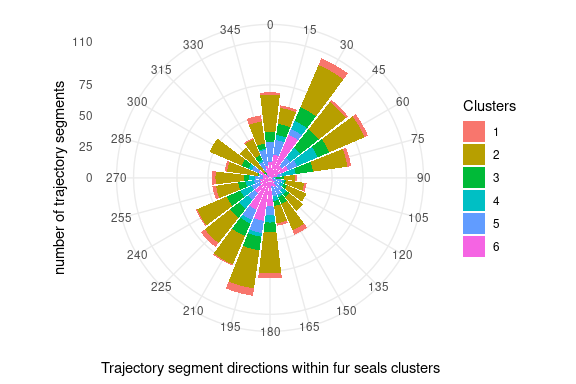## 4. Ontogenic stable isotope trajectories of juvenile fishes

In this section, we illustrate how to calculate trajectory metrics to characterize the ontogenic stable isotope trajectories of juvenile fishes. In the following sections, we show how to use these metrics as data to create a trajectory diagram.

We begin by loading the package dataset pike:

data("pike")

This is a data set from :

• Cucherousset, J., Paillisson, J.-M., Roussel, J.-M., 2013. Natal departure timing from spatially varying environments is dependent of individual ontogenetic status. Naturwissenschaften 100, 761–768.

Briefly, Cucherousset et al. (2013) released 192 individually tagged, hatchery-raised, juvenile pike (Esox lucius L.) with variable initial trophic position (fin $$\delta13C/\delta15N$$ values). Based on $$\delta15N$$ values, individuals were classified into zooplanktivorous ($$\delta15N$$ < 10 ‰) and piscivorous ($$\delta15N$$ > 10 ‰) as cannibalism is commonly observed in this species. Individuals were released in a temporarily flooded grassland where pike eggs usually hatch of the Brière marsh (France) to identify the determinants of juvenile natal departure. The release site was connected through a unique point to an adjacent pond used as a nursery habitat. Fish were continuously recaptured when migrating from flooded grassland to adjacent pond. Recaptured individuals (n = 29) were anaesthetized, checked for tags, measured for fork length, fin-clipped to quantify changes in $$\delta13C$$ and $$\delta15N$$ values, and released.

### 4.2 Calculating trajectory metrics and identification of trajectory clusters

First, we calculate net changes relative to the initial state for each individual (i.e. the distance between stable isotope compositions at release and at recapture):

Net_changes<-trajectoryLengths2D(pike[,7:8],pike$ID,pike$Time, relativeToInitial=TRUE)
colnames(Net_changes)<-c("Net_changes", "Trajectory")
pike$Net_Changes<-Net_changes$Net_changes

Then, we can use function hclust() to conduct a hierarchiacl clustering on the symmetric matrix D:

D=dist(pike[,7:8])
Ds<-trajectoryDistances(D,pike$ID, surveys = NULL, distance.type = "DSPD", symmetrization = "mean", add = TRUE, verbose = FALSE) We cut the dendrogram at height Hst to obtain a vector of cluster membership and copy it in pike: Hst=3 colstd<-c("black","yellow","green","blue","grey","red") hsxy <- hclust(Ds, "ward.D2") plot(hsxy,hang = -1, main="distance Pike", cex=.6) x<-rect.hclust (hsxy, h=Hst, border = colstd)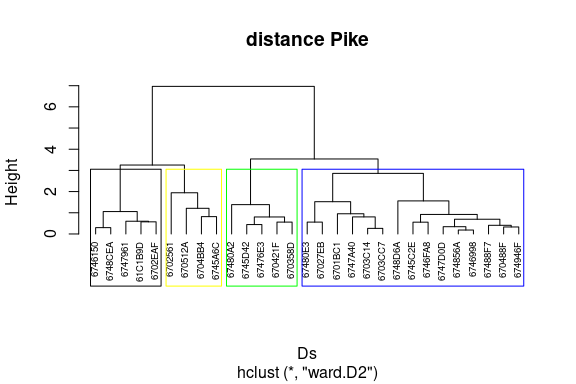# Store clusters into a new data column pike$Cluster<-cutree(hsxy, h=Hst)

### 4.3 Trajectory diagram of pike released in the flooded grassland and recaptured when emigrating into the adjacent pond

We prepare the data set to compute trajectory diagrams and density curves:

Pike1<-pike[pike$Time %in% 1,] Pike1<-Pike1[order(Pike1$ID, decreasing=FALSE),]
Pike1$Net_changes<-0 Pike2<-pike[pike$Time %in% 2,]
Pike2<-Pike2[order(Pike2$ID, decreasing=FALSE),] Pike2$Net_changes<-Net_changes$Net_changes data<-as.data.frame(rbind(Pike1,Pike2)) We create the trajectory diagram. Arrows represent trajectory path for each pit-tagged individual. Colors correspond to trajectory clusters. The dashed line separates piscivorous from zooplanktivorous individuals [zooplanktivorous ($$\delta15N$$ < 10) vs piscivorous ($$\delta15N$$ > 10)]. ggplot(data=data,aes(x=d13C,y=d15N,shape=Trophic_status_initial))+ geom_point(aes(size=Net_changes))+ geom_path(aes(x=d13C,y=d15N,group=ID,color=factor(Cluster)),arrow = arrow(length = unit(0.30, "cm")))+ geom_hline(yintercept=10, linetype="dashed", color = "black")+ xlab(expression(delta^13*"C")) + ylab(expression(delta^15*"N"))+ theme_minimal()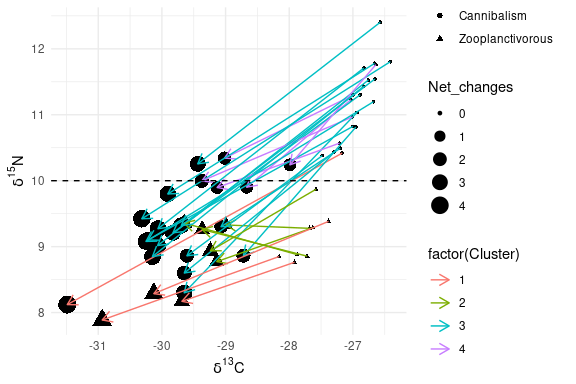Density curves X represents the distribution of all samples according to $$\delta13C$$ values, and capture (green=release; red=departure): gg_dist_d13C = ggplot(data, aes(d13C, fill=TimeL)) + geom_density(alpha=.5) gg_dist_d13C = gg_dist_d13C + ylab(expression(delta^13*"C"*" density")) gg_dist_d13C = gg_dist_d13C + theme(axis.title.y=element_blank(), axis.text=element_blank(), axis.line=element_blank(), axis.ticks=element_blank(), panel.grid.major = element_blank(), panel.grid.minor = element_blank(), panel.background =element_blank()) gg_dist_d13C = gg_dist_d13C + theme(legend.position = "none") gg_dist_d13C + scale_x_continuous(limits = c(-33, -25))+scale_y_continuous(limits = c(0, 1))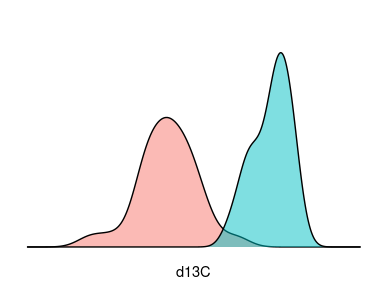Density curves Y represents the distribution of all samples according to $$\delta15N$$ values, and capture (green=release; red=departure): gg_dist_d15N = ggplot(data, aes(d15N, fill=TimeL)) + geom_density(alpha=.5) gg_dist_d15N = gg_dist_d15N + ylab(expression(delta^15*"N"*" density")) gg_dist_d15N =gg_dist_d15N gg_dist_d15N =gg_dist_d15N + coord_flip() gg_dist_d15N =gg_dist_d15N + theme(axis.title.y=element_blank(), axis.text=element_blank(), axis.line=element_blank(), axis.ticks=element_blank(), panel.grid.major = element_blank(), panel.grid.minor = element_blank(), panel.background =element_blank()) gg_dist_d15N =gg_dist_d15N +theme(legend.position = "none") gg_dist_d15N + scale_x_continuous(limits = c(7, 14))+scale_y_continuous(limits = c(0, 1))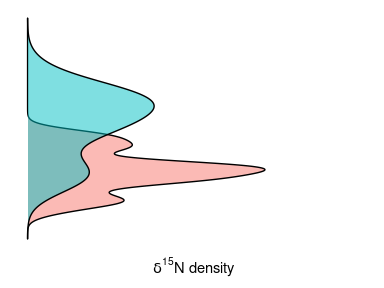## 5. Spatio-temporal variability of $$\delta 13C$$ and $$\delta 15N$$ modelled isoscapes in the Northeast Pacific In this section, we illustrate how to use trajectory metrics to characterize the spatio-temporal variability of $$\delta 13C$$ and $$\delta 15N$$ modelled isoscapes in the Northeast Pacific in an isoscape trajectory map and a trajectory heat map The datasets used in this application come from: • Espinasse, B., Hunt, B.P.V., Batten, S.D., Pakhomov, E.A., 2020. Defining isoscapes in the Northeast Pacific as an index of ocean productivity. Global Ecol Biogeogr 29, 246–261. Briefly, Espinasse et al. (2020) tested the application of isoscapes modelled from satellite data to the description of secondary production in the Northeast pacific. The output model fits in a 0.25° x 0.25° spatial grid covering the region spanning from 46 to 62°N and from 195 to 235°E and supporting $$\delta13C$$ and $$\delta15N$$ isoscapes from 1998 to 2017. We subset modelled $$\delta13C$$ and $$\delta15N$$ values of a 1° x 1° spatial grid from the original modelled dataset. Isoscapes modelled for 2013, 2015 and 2017 were selected as they were characterised by high stable isotope dynamics and consequently constitutes relevant inputs to test our isoscape trajectory map concept. Additionally, a long-term SITA analysis was performed from 1998 to 2017 using directions and net changes calculated for all pairs of dates (1998-1999,… ,2016-2017) as input for a trajectory heat map. ### 5.1 2013-2015 Isoscape trajectory maps We begin by loading the package dataset isoscape: data("isoscape") We calculate Segment lengths and Angle $$\alpha$$ for each stations to assess, respectively, the magnitude and the nature of change in the stable isotope space between 2013 and 2015. We then prepare a data set to compute the isoscape trajectory map with notably: Latitude, Longitude, Years, Angles and segment lenghts values: sites<-isoscape$station
surveys<-isoscape$Year Angl<-trajectoryAngles2D(isoscape[,3:4],sites,surveys, betweenSegments = FALSE) Length<-trajectoryLengths2D(isoscape[,3:4],sites,surveys) data<-as.data.frame(cbind(isoscape[1:489,],Angl,Length[,1])) colnames(data)<-c("Latitude","Longitude","d13C","d15N","Stations","Years","Angles","Lengths") head(data) ## Latitude Longitude d13C d15N Stations Years Angles Lengths ## 1 46 195 -24.24369 5.267485 1 2013 210.45063 0.31556226 ## 2 48 195 -24.32212 4.973764 3 2013 279.70681 0.28077863 ## 3 49 195 -24.28151 5.113458 4 2013 188.55168 0.03490337 ## 4 50 195 -24.30105 5.261582 5 2013 147.84429 0.25570108 ## 5 51 195 -24.05084 5.302065 6 2013 126.14883 0.32439073 ## 6 52 195 -23.92968 5.407169 7 2013 60.21061 0.28423495 Angles $$\alpha$$ need to be transformed for the use with geom_spoke. We then add transformed values in a new column Angles2. angle<-data$Angles
Angles2<-c()
for (i in 1:length(angle)) {
Angles2[i] <- c(ifelse(angle[i]==0,(angle[i]-270)*pi/180,
ifelse(angle[i]==180,(angle[i]-270)*pi/180,
ifelse(angle[i]==90,(angle[i]+270)*pi/180,
ifelse(angle[i]==270,(angle[i]+270)*pi/180,
ifelse(angle[i]==360,(angle[i]-270)*pi/180,
ifelse(angle[i]>0 & angle[i]<90 ,(90-angle[i])*pi/180,
ifelse(angle[i]>90 & angle[i]<180 ,(90-angle[i])*pi/180,
ifelse(angle[i]>180 & angle[i]<270,(180+(270-angle[i]))*pi/180,
ifelse(angle[i]>270 & angle[i]<360,(90+(360-angle[i]))*pi/180,"ERROR"))))))))))
}

data$Angles2<-Angles2 We now create the Isoscape trajectory map. In this kind of map, Segment lengths and Angles $$\alpha$$ are mapped to illustrate stable isotope spatio-temporal dynamics. Direction of arrows (angle $$\alpha$$) illustrate direction (i.e. the nature of change) in the modelled 2D $$\Omega \delta$$ space according to increase and/or decrease in $$\delta13C$$ and $$\delta15N$$ values (0-90°: + $$\delta13C$$ and + $$\delta15N$$; 90-180°: + $$\delta13C$$ and - $$\delta15N$$; 90-180°: + $$\delta13C$$ and - $$\delta15N$$; 180-270°: + $$\delta13C$$ and - $$\delta15N$$). Length of arrows and colored background rasters illustrate modelled trajectory segment length at each station (i.e. the magnitude of change). ggplot(data, aes(x = Longitude, y = Latitude, fill = Lengths, angle = Angles2, radius = rescale(Lengths, c(0.3, 1)))) + geom_raster(interpolate = TRUE) + geom_spoke(arrow = arrow(length = unit(.07, 'inches'))) + scale_fill_distiller(palette = "RdYlBu") + coord_equal(expand = 0) + theme(legend.position = 'bottom', legend.direction = 'horizontal', panel.background = element_rect(fill = "white"))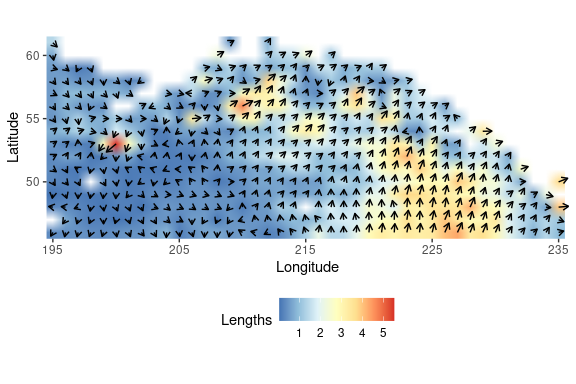### 5.2 1998-2017 trajectory heatmap We begin by loading the package dataset heatmapdata: data("heatmapdata") heatmapdata is composed of trajectory metrics for all stations within all inter-annual consecutive periods between 1998 and 2017: head(heatmapdata) ## Latitude Longitude d13C d15N Stations Years Angles Lengths ## 1 46 195 -24.76366 5.451872 1 1998-1999 181.31874 0.30196921 ## 2 47 195 -24.75540 5.334980 2 1998-1999 213.76898 0.09285079 ## 3 48 195 -24.51122 5.382608 3 1998-1999 183.00600 0.24017740 ## 4 49 195 -24.46314 5.481623 4 1998-1999 169.30735 0.34248078 ## 5 50 195 -24.27419 5.893179 5 1998-1999 174.57293 0.81250282 ## 6 51 195 -24.19454 5.780701 6 1998-1999 13.28445 0.96866737 ## Angles2 ## 1 4.689373 ## 2 4.123009 ## 3 4.659924 ## 4 -1.384174 ## 5 -1.476076 ## 6 1.338939 We then prepare the data set to create the trajectory heat map. We create a vector of direction, ranging by 15° between 0 and 360°, to class Angle $$\alpha$$ values. We create the vector ISPattern to characterize the pattern of direction according to changes in both stable isotope values (0-90°: + $$\delta13C$$ and + $$\delta15N$$; 90-180°: + $$\delta13C$$ and - $$\delta15N$$; 90-180°: + $$\delta13C$$ and - $$\delta15N$$; 180-270°: + $$\delta13C$$ and - $$\delta15N$$): #direction range deg <- 15 dir.breaks <- c(0,15,30,45,60,75,90,105,120,135,150,165,180,195,210,225,240,255,270,285,300,315,330,345,360) dir.binned <- cut(heatmapdata$Angles,
breaks = dir.breaks,
ordered_result = TRUE)

# bar labels
dir.labels <- as.character(c(seq(0, 360-deg, by = deg),0))

levels(dir.binned) <- dir.labels

heatmapdata$dir.binned <- dir.binned data<-heatmapdata[,c(6,7,8,10)] #direction vs SI patterns data<-data[order(data$dir.binned, decreasing=FALSE),]
rownames(data)<-1:9206
data$ISpattern<- c(rep("+d13C/+d15N",2862),rep("+d13C/-d15N",1840),rep("-d13C/-d15N",2931), rep("-d13C/+d15N",1573)) data1<-as.data.frame(table(data$dir.binned,data$Years)) data2<-aggregate(x = data$Lengths, by = list(data$dir.binned, data$Years), FUN=sum, drop=FALSE)
data2[is.na(data2)] <- 0

data1$Lengths<-data2$x
dfa<-data1
colnames(dfa)<-c("Directions","Periods","Nb_stations","Lengths")

The final dataset used to create the trajectory heat map is composed of four variables (“Directions”,“Periods”,“Nb_stations”,“Lengths”):

head(dfa)
##   Directions   Periods Nb_stations   Lengths
## 1          0 1998-1999          12  8.634497
## 2         15 1998-1999           9 11.096406
## 3         30 1998-1999           7  7.927697
## 4         45 1998-1999           8 11.138909
## 5         60 1998-1999           4  1.861980
## 6         75 1998-1999           4  1.835733

We plot trajectory metrics with respect to period and direction in a trajectory heat map. Angles $$\alpha$$ in the modelled 2D $$\Omega \delta$$ space exhibited by all stations within all pairs of dates (1998-1999,…,2016-2017) are represented by range of direction (15°) according to period. Color gradient from dark blue to yellow indicate the number of stations exhibited by a given range of direction within a given period.

ggplot(dfa, aes(Periods, Directions, fill= Nb_stations)) +
geom_tile() +
scale_fill_viridis(discrete=FALSE) +
theme_minimal()+
theme(axis.text.x = element_text(size=10, angle=90))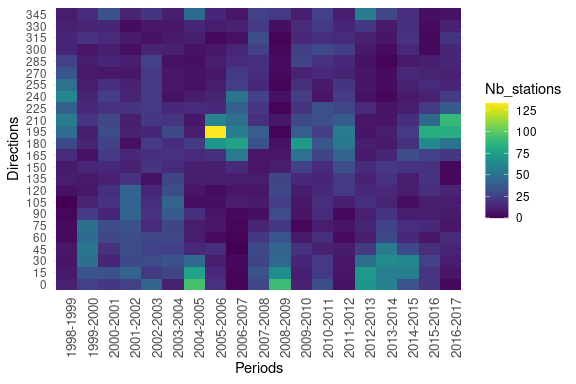The X bar plot represents the sum of segment lengths across stations and times, 1231 exhibiting the chosen angle. The blue gradient indicates the net change magnitude.

df.Xbarplot<-aggregate(dfa$Lengths, by = list(dfa$Periods), FUN = sum)
colnames(df.Xbarplot)<-c("Periods","Lengths")
bp.x <- ggplot(data = df.Xbarplot, aes(x = factor(Periods), y = Lengths)) +
geom_bar(stat = "identity", aes(fill = Lengths)) + theme_minimal() +
theme(axis.text.x = element_text(size = 10,angle=90),
axis.title.x = element_text(size = 20, margin = margin(10,0,0,0))) +
labs(x = "Periods")
bp.x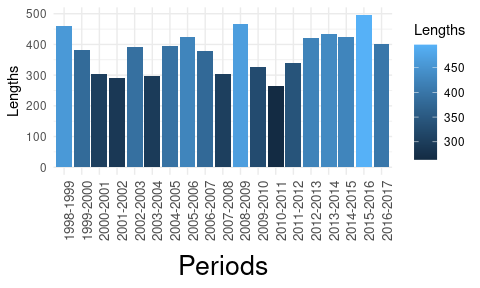The Y bar plot represents the overall net changes according to range of directions (angle $$\alpha$$). Bars are colored according to increase and/or decrease in $$\delta13C$$ and $$\delta15N$$ values (Pink: 0-90°: + $$\delta13C$$ and + $$\delta15N$$ ; Blue: 90-180°: + $$\delta13C$$ and - $$\delta15N$$; Red: 90-180°: + $$\delta13C$$ and - $$\delta15N$$; Green: 180-270°: + $$\delta13C$$ and - $$\delta15N$$).

df.Ybarplot<-aggregate(dfa$Lengths, by = list(dfa$Directions), FUN = sum)
colnames(df.Ybarplot)<-c("Directions","Lengths")
df.Ybarplot\$ISpattern<- c(rep("+d13C/+d15N",6),rep("+d13C/-d15N",6),rep("-d13C/-d15N",6), rep("-d13C/+d15N",6))

bp.y <- ggplot(data = df.Ybarplot, aes(x = factor(Directions), y = Lengths,fill = ISpattern)) +
geom_bar(stat="identity") + theme_minimal() + coord_flip()
bp.y